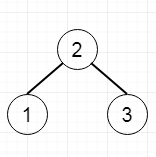# Sum Root to Leaf Numbers in Python

Suppose we have a binary tree containing digits from 0-9 only, here all root-to-leaf path could represent a number.

So if the tree is like −This is representing two paths 21 and 23, so the output will be 21 + 23 = 44.

To solve this, we will follow these steps −

• Create one recursive function called dfs(), this will take root, and num. initially num = 0
• if the node is not null
• num := num * 10 + value of node
• if node right is not null and node left is not null, then’
• sum := sum + num
• num := num / 10
• return from the function
• dfs(right of node, num)
• dfs(left of node, num)
• num := num / 10
• return from the method
• initially sum := 0
• call the dfs using root, and
• return sum.

## Example(Python)

Let us see the following implementation to get a better understanding −

Live Demo

class TreeNode:
def __init__(self, data, left = None, right = None):
self.data = data
self.left = left
self.right = right
def insert(temp,data):
que = []
que.append(temp)
while (len(que)):
temp = que
que.pop(0)
if (not temp.left):
if data is not None:
temp.left = TreeNode(data)
else:
temp.left = TreeNode(0)
break
else:
que.append(temp.left)
if (not temp.right):
if data is not None:
temp.right = TreeNode(data)
else:
temp.right = TreeNode(0)
break
else:
que.append(temp.right)
def make_tree(elements):
Tree = TreeNode(elements)
for element in elements[1:]:
insert(Tree, element)
return Tree
class Solution(object):
def sumNumbers(self, root):
self.sum = 0
self.dfs(root)
return self.sum
def dfs(self,node,num=0):
if node:
num = num*10 + node.data
if not node.right and not node.left:
self.sum+=num
num/=10
return
self.dfs(node.right,num)
self.dfs(node.left,num)
num/=10
return
ob1 = Solution()
tree = make_tree([2,1,3])
print(ob1.sumNumbers(tree))

## Input

[2,1,3]

## Output

44